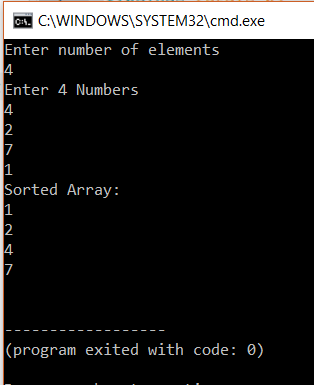AWS Global Infrastructure

### Programming & Frameworks

Topics Covered
• C Programming and Data Structures (41 Blogs)
• Comprehensive Java Course (2 Blogs)
• Java/J2EE and SOA (324 Blogs)
• Spring Framework (8 Blogs)
SEE MORE

# How To Implement Selection Sort in C?

Published on Jul 15,2019 7.7K ViewsHow To Implement Selection Sort in C?

In the previous article we have discussed the implementation of Bubble Sort in C. This article will brief you on how to implement Selection Sort in C. Following are the pointers this article will focus on,

Let us get started with it then,

## Selection Sort in C

Selection sort is another algorithm that is used for sorting. This sorting algorithm, iterates through the array and finds the smallest number in the array and swaps it with the first element if it is smaller than the first element. Next, it goes on to the second element and so on until all elements are sorted.

Example of Selection Sort

Consider the array:

[10,5,2,1]

The first element is 10. The next part we must find the smallest number from the remaining array. The smallest number from 5 2 and 1 is 1. So, we replace 10 by 1.

The new array is [1,5,2,10] Again, this process is repeated.

Finally, we get the sorted array as [1,2,5,10].

Let us continue with this article on Selection Sort in C and see how the algorithm works,

Algorithm for Selection Sort:

Step 1 − Set min to the first location

Step 2 − Search the minimum element in the array

Step 3 – swap the first location with the minimum value in the array

Step 4 – assign the second element as min.

Step 5 − Repeat the process until we get a sorted array.

Let us take a look at the code for the the programmatic implementation,

Code for Selection Sort:

```
#include <stdio.h>
int main()
{
int a, n, i, j, position, swap;
printf("Enter number of elementsn");
scanf("%d", &n);
printf("Enter %d Numbersn", n);
for (i = 0; i < n; i++)
scanf("%d", &a[i]);
for(i = 0; i < n - 1; i++)
{
position=i;
for(j = i + 1; j < n; j++)
{
if(a[position] > a[j])
position=j;
}
if(position != i)
{
swap=a[i];
a[i]=a[position];
a[position=swap;
}
}
printf("Sorted Array:n");
for(i = 0; i < n; i++)
printf("%dn", a[i]);
return 0;
}

```

Output:In the above program, we first take the number of terms from the user and store it in n. Next, the user needs to enter the array. The array is accepted and stored in a[].

The first ‘for loop’ takes care of the element to be matched. It assigns i to the position variable. The inner ‘for loop’ is used to iterate through the remaining elements and find the smallest element. Once the smallest element is found the j is assigned to the position variable. j holds the index of the smallest element.

Then, it is checked if the position variable is not equal to i. If it is not equal, swapping takes place, using a swap variable. Let us now move to the final bit of this article on Selection Sort in C,

Other ways to implement Selection Sort:

There are other ways to do the selection sort. One such way is by using a sort function. In this program, we will do the sort in the function. Remaining aspects of the program will be the same.

Here is the code:

Output:

```
#include <stdio.h>
void SelSort(int array[],int n);
int main()
{
int array, n,i;
printf("Enter number of elementsn");
scanf("%d", &n);
printf("Enter %d Numbersn", n);
for(i = 0; i < n; i++)
scanf("%d", &array[i]);
SelSort(array,n);
return 0;
}
void SelSort(int array[], int n)
{
int i, j, position, swap;
for(i = 0; i < (n - 1); i++)
{
position=i;
for(j = i + 1; j < n; j++)
{
if(array[position]>array[j])
position=j;
}
if(position != i)
{
swap=array[i];
array[i]=array[position];
array[position]=swap;
}
}
printf("Sorted Array:n");
for(i = 0; i < n; i++)
printf("%dn", array[i]);
}

```

The execution of the program is the same, but the only difference is that in this program a function is called to do the sorting.

Selection Sort is done in this manner.

With this we come to the end of this blog on ‘Selection Sort In C’. I hope you found this informative and helpful, stay tuned for more tutorials on similar topics.You may also checkout our training program to get in-depth knowledge on jQuery along with its various applications, you can enroll here for live online training with 24/7 support and lifetime access.

Got a question for us? Mention them in the comments section of  this blog and we will get back to you.REGISTER FOR FREE WEBINARThank you for registering Join Edureka Meetup community for 100+ Free Webinars each month